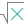#LOG (PQL - xl)

This function returns the logarithm base Y of a number X

• Library: PQL \ Spreadsheet \ Math & Trig
• Compatibility: Any content (regardless of data source) in the Tabulate spreadsheet module
• Solve: This function can be used with the Solve plug-in: both as part of the objective function and constraint functions

#### Syntax

LOG(number, OPTIONAL base)

##### Function Arguments
 Name Description Type Optional number Number for which to find a logarithm. This must be a positive real number Number base Base of the logarithm; if omitted, the calculation uses 10 as the base (See LOG10.) Number Y